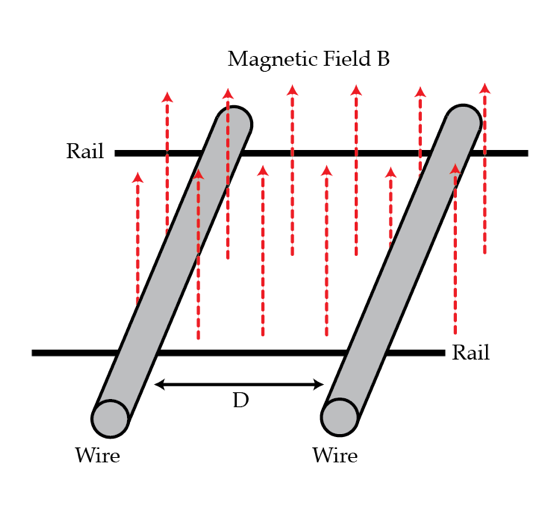# Superconducting rails and magnetic friction

Two identical wires with resistance $R$ and mass $m$ rest on two smooth parallel superconducting (zero resistance) rails. Initially, they are separated by a distance $D=70~\mbox{cm}$. Suddenly, a uniform magnetic field $B$, perpendicular to the plane of the rails is switched on and the wires start rolling. What will be the final distance in centimeters between the wires? Assume that the wires are thin and that the process of switching on the magnetic field happens very fast.×